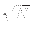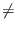Next: Operator to Create Equal Up: Grover's Algorithm Previous: An Illustration of Grover's   Contents

## Outline of Proof of Correctness of Grover's Algorithm

To prove that Grover's algorithm successfully finds the unique marked state in O() operations we must show the following:

1. That there is a operator to produce a equal superposition of states for part 1 of the algorithm. This operation is well known and referred to as the Walsh-Hadamard operator.

2. That there is a operator to rotate the phase of a given state.

3. That the definition of the matrix A : Aij = 2/N if ij and Aii = - 1 + 2/N is an inversion about average operator.

4. That the matrix representations of all operators used are unitary. If this is the case then these transformations are physically realizable.

5. That repeated applications of step 2 of the algorithm increase the amplitude of the marked state, such that after O() iterations the probability of measuring the marked state is at least 1/2.Next: Operator to Create Equal Up: Grover's Algorithm Previous: An Illustration of Grover's   Contents
Matthew Hayward - Quantum Computing and Grover's Algorithm GitHub Repository